Courses

# Residues in Complex Analysis - Mathematical Methods of Physics, UGC - NET Physics Physics Notes | EduRev

## Physics : Residues in Complex Analysis - Mathematical Methods of Physics, UGC - NET Physics Physics Notes | EduRev

The document Residues in Complex Analysis - Mathematical Methods of Physics, UGC - NET Physics Physics Notes | EduRev is a part of the Physics Course Physics for IIT JAM, UGC - NET, CSIR NET.
All you need of Physics at this link: Physics

In mathematics, more specifically complex analysis, the residue is a complex number proportional to the contour integral of a meromorphic function along a path enclosing one of its singularities. (More generally, residues can be calculated for any function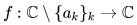that is holomorphic except at the discrete points {ak}k, even if some of them are essential singularities.) Residues can be computed quite easily and, once known, allow the determination of general contour integrals via the residue theorem

Definition

The residue of a meromorphic function f at an isolated singularity {\displaystyle a}, often denoted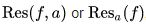, is the unique value R such that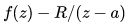has an analyticantiderivative in a punctured disk . 0 < |z - a| < δ

Alternatively, residues can be calculated by finding Laurent series expansions, and one can define the residue as the coefficient a−1 of a Laurent series.

The definition of a residue can be generalized to arbitrary Riemann surfaces. Suppose ω is a 1-form on a Riemann surface. Let ω be meromorphic at some point x, so that we may write ω in local coordinates as  f(z) dz Then the residue of ω at x is defined to be the residue of  f(z) at the point corresponding to x.

Examples

Residue of a monomial

Computing the residue of a monomial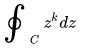makes most residue computations easy to do. Since path integral computations are homotopy invariant, we will let C be the circle with radius 1. Then, using the change of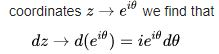hence our integral now reads as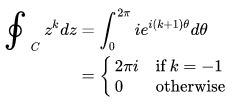Application of monomial residue

As an example, consider the contour integral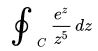where C is some simple closed curve about 0.

Let us evaluate this integral using a standard convergence result about integration by series. We can substitute the Taylor series for ze into the integrand. The integral then becomes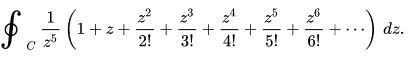Let us bring the 1/z5 factor into the series. The contour integral of the series then writes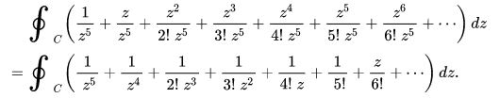Since the series converges uniformly on the support of the integration path, we are allowed to exchange integration and summation. The series of the path integrals then collapses to a much simpler form because of the previous computation. So now the integral around C of every other term not in the form cz−1 is zero, and the integral is reduced to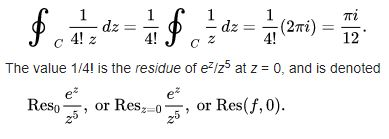Calculating residues

Suppose a punctured disk D = {z : 0 < |z − c| < R} in the complex plane is given and f is a holomorphic function defined (at least) on D. The residue Res(fc) of f at c is the coefficient a−1 of (z − c)−1 in the Laurent series expansion of f around c. Various methods exist for calculating this value, and the choice of which method to use depends on the function in question, and on the nature of the singularity.

According to the residue theorem, we have: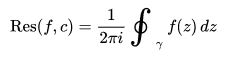where γ traces out a circle around c in a counterclockwise manner. We may choose the path γ to be a circle of radius ε around c, where ε is as small as we desire. This may be used for calculation in cases where the integral can be calculated directly, but it is usually the case that residues are used to simplify calculation of integrals, and not the other way around.

Removable singularities

If the function f can be continued to a holomorphic function on the whole disk { |yc| < R }, then Res(fc) = 0. The converse is not generally true.

Simple poles

At a simple pole c, the residue of f is given by: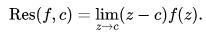It may be that the function f can be expressed as a quotient of two functions, f(z)=g(z)/h(z), where g and h are holomorphic functions in a neighbourhood of c, with h(c) = 0 and h'(c) ≠ 0. In such a case, L'Hôpital's rule can be used to simplify the above formula to: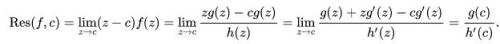Limit formula for higher order poles

More generally, if c is a pole of order n, then the residue of f around zc can be found by the formula: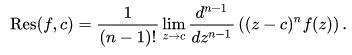This formula can be very useful in determining the residues for low-order poles. For higher order poles, the calculations can become unmanageable, and series expansion is usually easier. For essential singularities, no such simple formula exists, and residues must usually be taken directly from series expansions.

Residue at infinity

In general, the residue at infinity is given by: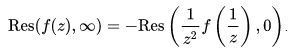If the following condition is met: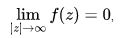then the residue at infinity can be computed using the following formula: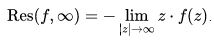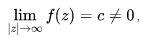then the residue at infinity is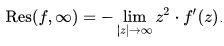Series methods

If parts or all of a function can be expanded into a Taylor series or Laurent series, which may be possible if the parts or the whole of the function has a standard series expansion, then calculating the residue is significantly simpler than by other methods.

1. As a first example, consider calculating the residues at the singularities of the function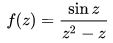which may be used to calculate certain contour integrals. This function appears to have a singularity at z = 0, but if one factorizes the denominator and thus writes the function as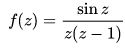it is apparent that the singularity at z = 0 is a removable singularity and then the residue at z = 0 is therefore 0.

The only other singularity is at z = 1. Recall the expression for the Taylor series for a function g(z) about za: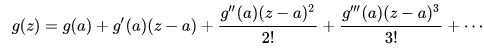So, for g(z) = sin z and a = 1 we have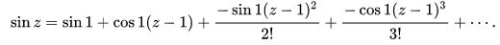and for g(z) = 1/z and a = 1 we have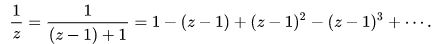Multiplying those two series and introducing 1/(z − 1) gives us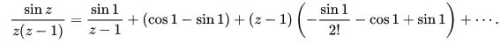So the residue of f(z) at z = 1 is sin 1.

2. The next example shows that, computing a residue by series expansion, a major role is played by the Lagrange inversion theorem. Let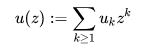be an entire function, and let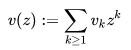with positive radius of convergence, and with  ν1 ≠ 0. So  V(z) has a local inverse V(z) at 0, and u(1/ V(z))  is meromorphic at 0. Then we have: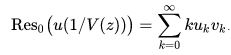Indeed,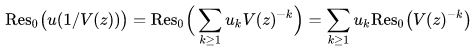because the first series converges uniformly on any small circle around 0. Using the Lagrange inversion theorem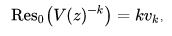and we get the above expression. For example, if u(z) = z + z2 and also v(z) = z + z2, then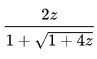and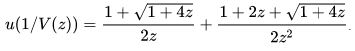The first term contributes 1 to the residue, and the second term contributes 2 since it is asymptotic to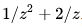Note that, with the corresponding stronger symmetric assumptions on u(z) and v(z) , it also follows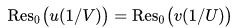where U(z)  is a local inverse of u(z)  at 0.

Offer running on EduRev: Apply code STAYHOME200 to get INR 200 off on our premium plan EduRev Infinity!

159 docs

,

,

,

,

,

,

,

,

,

,

,

,

,

,

,

,

,

,

,

,

,

,

,

,

;